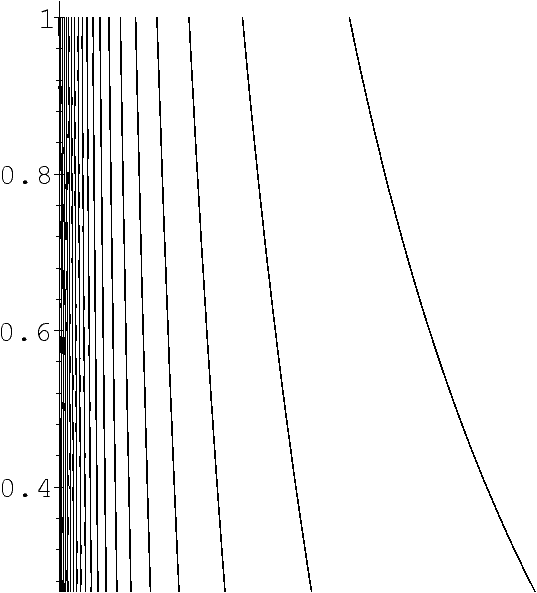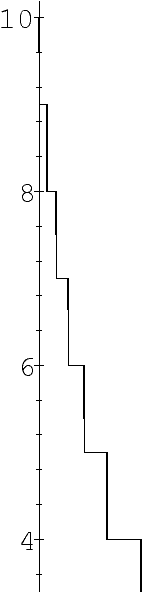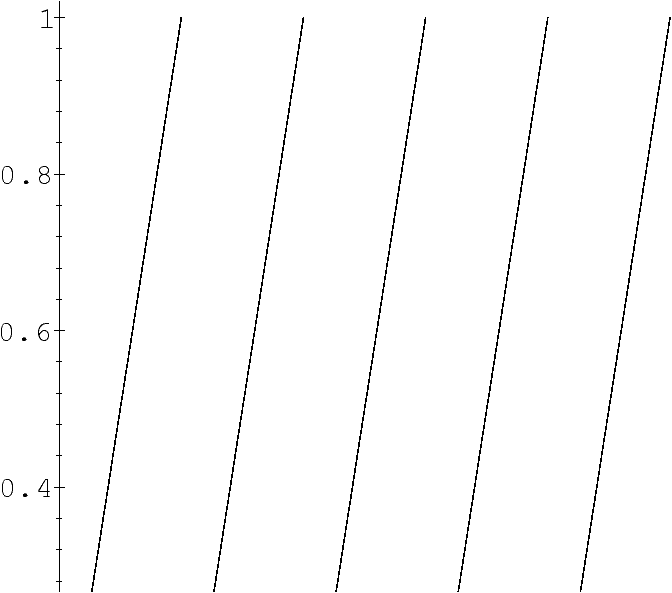Dynamical Systems and Average-Case Analysis of General Tries

Brigitte Vallée

GREYC, Université de Caen, France

Algorithms Seminar

June 9, 1997

[summary by Julien Clément]

A properly typeset version of this document is available in postscript and in pdf.

If some fonts do not look right on your screen, this might be fixed by configuring your browser (see the documentation here).

Abstract
The three major parameters of a trie (sometimes called digital tree), number of nodes, path length, and height, are analyzed precisely in a general context where words are emitted by a source associated to a dynamical system. The results can all be stated in terms of two intrinsic characteristics of the source: the entropy and the probability of letter coincidence. These characteristics themselves are linked in a natural way to spectral properties of a Ruelle operator associated to the dynamical system.

## 1   Probabilistic dynamical sources

### 1.1   Definitions

A dynamical source, in the context of information theory, is a mechanism which produces infinite words over an alphabet M. Such a system is defined by four elements: (i) an alphabet M included in N, (ii) a quasi partition of I=]0,1[ with intervals Im, m Î M, (iii) a mapping s: I ® M which is constant over each Im and equal to m and finally (iv) a mapping T: I ® I which satisfies two properties: the restriction of T to Im is a real analytic bijection from Im to I; the mapping T is expansive, i.e. |T'(x)|>1 on I. The words emitted by the source are produced by iterating T and coded thanks to s. The word M(x) of M¥ (an infinite sequence of symbols), where xÎ I, is formed with the symbols
M(x):=(s(x),s(T(x)),s(T2(x)),...,s(Tk(x)),...).
Each letter of the alphabet is associated to a distinct branch of T or equivalently to a distinct inverse branch of T denoted by hm. The bijection hm: I ® Im coincides with the inverse of the restriction of T to Im.

### 1.2   ExamplesFigure 1: Graphical representation of the mappings T and s for a source based on the continued fraction expansion (left) and for a memoryless source based on the 5-ary expansion of numbers (right).

A lot of probabilistic dynamical sources can be described in such a framework, including all memoryless sources where letters of the alphabet can be emitted with probabilities {pi} independently of previous letters. This gives a mapping T where branches are affine. In particular this encompasses the b-ary expansion model of numbers. Markov sources take into account a finite past for producing words and thus generalize memoryless sources. Finally, in a model where the source is based upon the continued fraction expansion of numbers, the alphabet is N and the probability for a character to be emitted depends on all the previous history.

### 1.3   Fundamental intervals, entropy, coincidence probability

Numbers which share the same prefix expansion m1,...,mk form an interval called fundamental interval of depth k which is exactly, with the preceding notations,
I
 m1,...,mk
=h
 m1
° ... ° h
 mk
( I).
In a general context, the interval I is endowed with a continuous density f (so that F denotes the associated distribution). Then the word M(x) is produced according to the expansion process (using T and s). In this context, the measure uh of a fundamental interval Ih associated to h=hm1 ° ... ° hmk is
uh:= |F(h(0))-F(h(1))|,
and plays a crucial role since it is the probability that a word begins with a certain prefix. During the analysis, two quantities relative to the source appear naturally. The entropy h( S,F) and the coincidence probability c( S,F) are defined as the limits
h( S ,F):=
 lim k ® ¥
-1
k
 å |h|=k
uh log uh,     c( S ,F):=
 lim k ® ¥
é
ê
ê
ë
 å |h|=k
uh log uh ù
ú
ú
û
1/k.
It is interesting to note that these limits exist and are independent of the distribution F.

## 2   Tries associated to a general source

Let M Î N be a set of elements called digits, and M¥ the set of all infinite sequences built over M. For any word produced by the dynamical source M(x)=(s(x),s(Tx),s(T2x),...) (with x Î I), the head and tail functions are defined by
Any finite set of infinite words produced by the same source can be written as M(X)={M(x) |x Î X}, and one associates to X a trie, Trie(X), defined by the following recursive rules
• (R1) If X={x} has cardinality equal to 1, then Trie(X) consists of a single leaf node that contains M(x).
• (R2) If X has cardinality at least 2, then Trie(X) is an internal node represented generically by `o' to which are attached l subtrees, where l=card{s(x) |x Î X} is the number of different head symbols in M(X). Let b1<...< bl be these head symbols; Trie(X) is defined by
Trie(X)=á o,Trie(X1),...,Trie (X  l
)ñ,   where   Xj={Tx| s(x)=bj,   x Î X}.
The trie Trie(Xj) collects all the suffixes of words that begin with bj.

### 2.1   Parameters

The main parameters of a trie are size (number of internal nodes), height and external length path, which is the sum of all links from the root to each leaf.

The model considers here infinite strings emitted independently by the same dynamical source. Rather than considering a fixed number n of strings, a Poisson model of rate n is used, where the number of strings N is also a random variable which strongly concentrates around n. The strong property of independence of this particular model makes the analysis easier and gives access to the expectations of parameters. The expectations of height, size and external path length under a Poisson model of rate n and distribution F over I are respectively
D(n)=
 å k
[1-
 Õ |h|=k
(1+nuh)e
 -n uh
],   S(n)=
 å h
[1-(1+n uh) e
 -n uh
],   P(n)=
 å h
n uh [1-e
 -n uh
].

### 2.2   Asymptotics

These quantities are easily recognized as harmonic sums of the form F(x)=åk Î K lk fk x) (excepted for the height which needs a small calculation step before). The best tool to analyze the asymptotics of such harmonic sums is the Mellin transform, which leads to locating poles of the associated Dirichlet series L(s)=åk Î K lk µks. Here, the key quantity for the analysis of size and path length is the series of fundamental intervals,
L(F,s)=
 å h
uhs=
 å h
|F(h(0))-F(h(1))|s,
considered for complex values of s. For a general source, it is not always easy (or possible) to locate precisely the singularities. In this case a Tauberian theorem, under some constraints, can be used to extract the asymptotic expansion.

## 3   Generalized Ruelle operators

### 3.1   Presentation

The generalized Ruelle operators are derived from the original Ruelle operators, and involve secants of the inverse branches H(u,v):=|(h(u)-h(v))/(u-v)|. The generalized Ruelle operators are defined by
G s[F](u,v)=
 å |h|=1
 ~ H
(u,v)s F(h(u),h(s)),
where H~ is the analytic extension of H and s is a complex parameter. Here the sum is taken over branches of depth 1. If we define the secant L of the distribution F,
L(x,y)= ½
½
½
½
F(x)-F(y)
x-y
½
½
½
½
,
then the Dirichlet series can be expressed as
L(F,s)=
 å h
uhs=
 å h
|F(h(0))-F(h(1))|s= (I-Gs)-1[Ls](0,1).

### 3.2   Singularities of the quasi inverse (I-Gs)-1

Singularities of (I-Gs)-1 are of special interest because these are also singularities of L(F,s). These singularities arise for values of s where Gs has an eigenvalue equal to 1. In particular, there is always a pole at s=1 (easy to prove from the previous form of L(F,s)). One can derive from strong properties of the operator Gs that there are three different cases, called periodic, quasi-periodic and aperiodic, depending on the precise nature of the eigenvalues of Gs on the line Â(s)=1. The operator Gs has also special properties at s=1 and s=2 since the entropy of the source is h( S)=-l'(1) (the derivative of the dominant eigenvalue at s=1), while the coincidence probability is c( S)=l(2).

## 4   Average-case analysis of general tries

### 4.1   Analysis of height

The analysis of height is based on estimates of the individual probabilities pk(n)=Õ|h|=k (1+n uh) e-n uh followed by a Mellin analysis. This leads to the asymptotic expansion
D(n)=
2
| log c( S)|
log n +PF(log n)+ g+AF+o(1)

### 4.2   Analysis of size and path length

The operator (I-Gs)-1 has a simple pole at s=1, and thus gives the main term of the asymptotic expansion. For a general source, a Tauberian theorem can be applied to estimate the contribution of others poles. Finally one has
P(n)=
1
h( S)
n log n + o(n log n),     S(n)=
1
h( S)
n + o(n).

## 5   Some important particular cases

### 5.1   Bernoulli sources

The Bernoulli source considers a finite alphabet M={1,..., r} with probability of emission {p1,...,pr} (with p1+...+pr=1). In this case the entropy and the coincidence probability have classical expressions
H=-
 r å i=1
pi log pi,     c=
 r å i=1
pi2.

### 5.2   Continued fraction source

The continued fraction expansion of numbers can be considered as a dynamical source over the infinite alphabet M=N. The operator of Ruelle is then called the Ruelle-Mayer operator and is defined by
G s[f](z)=
 å m ³ 1
1
(m+z)s
f(
1
m+z
).
The entropy of the source is linked to the so-called Lévy's constant which plays a central rôle in the the analysis of the Euclidean algorithm. The coincidence probability is a constant which intervenes in two-dimensional generalizations of the Euclidean algorithm. These constants are
l'(1)=
p2
6 log 2
,   l(2) ~ 0.1994.

## References


Brigitte Vallée. -- Dynamical systems and average-case analysis of general tries. -- Les cahiers du GREYC, 1997.


Flajolet (Philippe) and Vallée (Brigitte). -- Continued Fraction Algorithms, Functional Operators, and Structure Constants. -- Research Report n°2931, Institut National de Recherche en Informatique et en Automatique, July 1996. 33 pages. Invited lecture at the 7th Fibonacci Conference, Graz, July 1996; to appear in Theoretical Computer Science.


Hervé Daudé (Philippe Flajolet) and Vallée (Brigitte). -- An Average-case Analysis of the Gaussian Algorithm for Lattice Reduction. -- Research Report n°2798, Institut National de Recherche en Informatique et en Automatique, February 1996. To appear in Combinatorics, Probability and Computing, 1997.


Jacquet (Philippe) and Szpankowski (Wojciech). -- Analysis of digital tries whith Markovian dependency. IEEE Transactions on Information Theory, vol. 37, n°5, September 1991, pp. 1470--1475.


Vallée (Brigitte). -- Opérateurs de Ruelle-Mayer généralisés et analyse en moyenne des algorithmes d'Euclide et de Gauss. Acta Arithmetica, vol. LXXXI, n°2, 1997, pp. 101--144.

This document was translated from LATEX by HEVEA.